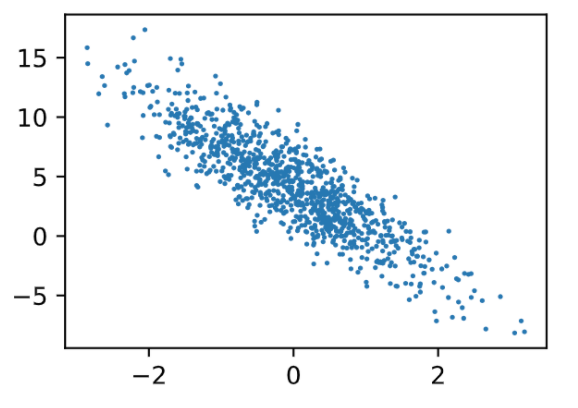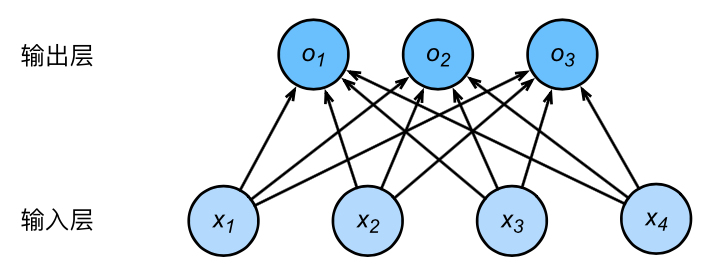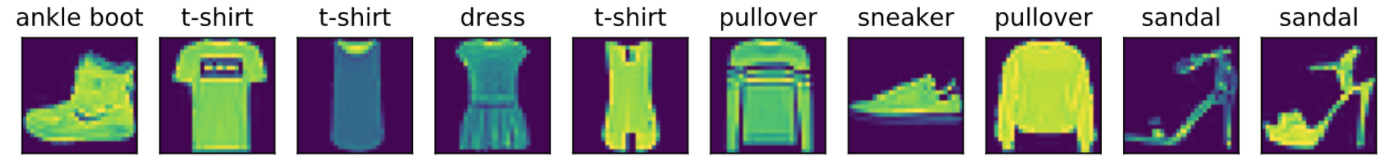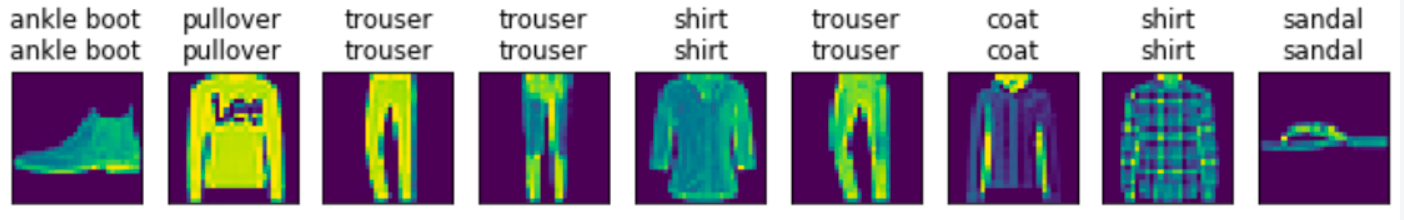# 第3章(3.2～3.7节)线性/Softmax回归实现【深度学习基础】--动手学深度学习【Tensorflow2.0版本】

UC 伯克利李沐的《动手学深度学习》开源书一经推出便广受好评。很多开发者使用了书的内容，并采用各种各样的深度学习框架将其复现。

# 3.2 线性回归的从零开始实现

%matplotlib inline
import tensorflow as tf
print(tf.__version__)
from matplotlib import pyplot as plt
import random


## 3.2.1 生成数据集

y = X w + b + ϵ \boldsymbol{y} = \boldsymbol{X}\boldsymbol{w} + b + \epsilon

num_inputs = 2
num_examples = 1000
true_w = [2, -3.4]
true_b = 4.2
features = tf.random.normal((num_examples, num_inputs),stddev = 1)
labels = true_w * features[:,0] + true_w * features[:,1] + true_b
labels += tf.random.normal(labels.shape,stddev=0.01)


print(features, labels)


tensor([0.8557, 0.4793]) tensor(4.2887)


def set_figsize(figsize=(3.5, 2.5)):
plt.rcParams['figure.figsize'] = figsize

set_figsize()
plt.scatter(features[:, 1], labels, 1)## 3.2.2 读取数据

import numpy as np
def data_iter(batch_size, features, labels):
features = np.array(features)
labels = np.array(labels)
num_examples = len(features)
indices = list(range(num_examples))
random.shuffle(indices)
for i in range(0, num_examples, batch_size):
j = np.array(indices[i:min(i + batch_size, num_examples)])
yield features[j], labels[j]


batch_size = 10

for X, y in data_iter(batch_size, features, labels):
print(X, y)
break


tensor([[-1.4239, -1.3788],
[ 0.0275,  1.3550],
[ 0.7616, -1.1384],
[ 0.2967, -0.1162],
[ 0.0822,  2.0826],
[-0.6343, -0.7222],
[ 0.4282,  0.0235],
[ 1.4056,  0.3506],
[-0.6496, -0.5202],
[-0.3969, -0.9951]])
tensor([ 6.0394, -0.3365,  9.5882,  5.1810, -2.7355,  5.3873,  4.9827,  5.7962,
4.6727,  6.7921])


## 3.2.3 初始化模型参数

w = tf.Variable(tf.random.normal((num_inputs, 1), stddev=0.01))
b = tf.Variable(tf.zeros((1,)))


## 3.2.4 定义模型

def linreg(X, w, b):
return tf.matmul(X, w) + b


## 3.2.5 定义损失函数

def squared_loss(y_hat, y):
return (y_hat - tf.reshape(y, y_hat.shape)) ** 2 /2


## 3.2.6 定义优化算法

def sgd(params, lr, batch_size,t,l):
for param in params:
#         param[:] = param - lr * t.gradient(l, param) / batch_size
param.assign_sub(lr * t.gradient(l, param) / batch_size)


## 3.2.7 训练模型

lr = 0.03
num_epochs = 3
net = linreg
loss = squared_loss

for epoch in range(num_epochs):
for X, y in data_iter(batch_size, features, labels):
t.watch([w,b])
l = loss(net(X, w, b), y)
sgd([w, b], lr, batch_size)
train_l = loss(net(features, w, b), labels)
print('epoch %d, loss %f' % (epoch + 1, tf.reduce_mean(train_l)))


epoch 1, loss 0.028127
epoch 2, loss 0.000095
epoch 3, loss 0.000050


print(true_w, '\n', w)
print(true_b, '\n', b)


[2, -3.4]
tensor([[ 1.9998],
4.2


## 小结

• 可以看出，仅使用VariablesGradientTape模块就可以很容易地实现一个模型。接下来，本书会在此基础上描述更多深度学习模型，并介绍怎样使用更简洁的代码（见下一节）来实现它们。

# 3.3 线性回归的简洁实现

## 3.3.1 生成数据集

import tensorflow as tf

num_inputs = 2
num_examples = 1000
true_w = [2, -3.4]
true_b = 4.2
features = tf.random.normal(shape=(num_examples, num_inputs), stddev=1)
labels = true_w * features[:, 0] + true_w * features[:, 1] + true_b
labels += tf.random.normal(labels.shape, stddev=0.01)


## 3.3.2 读取数据

from tensorflow import data as tfdata

batch_size = 10
# 将训练数据的特征和标签组合
dataset = tfdata.Dataset.from_tensor_slices((features, labels))
# 随机读取小批量
dataset = dataset.shuffle(buffer_size=num_examples)
dataset = dataset.batch(batch_size)
data_iter = iter(dataset)


shufflebuffer_size 参数应大于等于样本数，batch 可以指定 batch_size 的分割大小。

for X, y in data_iter:
print(X, y)
break

tf.Tensor(
[[ 1.2856768   1.3815335 ]
[ 1.1151928  -1.3777982 ]
[ 0.6097271   1.3478378 ]
[ 2.1615875   1.52963   ]
[-1.3143488  -0.79531455]
[-2.495006    0.3701927 ]
[-0.07739297 -0.8636043 ]
[-0.18479416 -1.5275241 ]
[-0.3426277  -0.01935842]
[ 0.25231913  1.4940815 ]], shape=(10, 2), dtype=float32) tf.Tensor(
[ 2.0673854  11.10116     0.8320709   3.3300133   4.272185   -2.062947
6.981174    9.027803    3.5848885  -0.39152586], shape=(10,),     dtype=float32)


for (batch, (X, y)) in enumerate(dataset):
print(X, y)
break


## 3.3.3 定义模型和初始化参数

Tensorflow 2.0推荐使用Keras定义网络，故使用Keras定义网络

Keras中，Sequential实例可以看作是一个串联各个层的容器。

Keras 中初始化参数由 kernel_initializerbias_initializer 选项分别设置权重和偏置的初始化方式。我们从 tensorflow 导入 initializers 模块，指定权重参数每个元素将在初始化时随机采样于均值为0、标准差为0.01的正态分布。偏差参数默认会初始化为零。RandomNormal(stddev=0.01)指定权重参数每个元素将在初始化时随机采样于均值为0、标准差为0.01的正态分布。偏差参数默认会初始化为零。

from tensorflow import keras
from tensorflow.keras import layers
from tensorflow import initializers as init
model = keras.Sequential()


## 3.3.4 定义损失函数

Tensoflowlosses模块中提供了各种损失函数和自定义损失函数的基类，并直接使用它的均方误差损失作为模型的损失函数。

from tensorflow import losses
loss = losses.MeanSquaredError()


## 3.3.5 定义优化算法

from tensorflow.keras import optimizers
trainer = optimizers.SGD(learning_rate=0.03)


## 3.3.6 训练模型

num_epochs = 3
for epoch in range(1, num_epochs + 1):
for (batch, (X, y)) in enumerate(dataset):
l = loss(model(X, training=True), y)

l = loss(model(features), labels)
print('epoch %d, loss: %f' % (epoch, l.numpy().mean()))

epoch 1, loss: 0.519287
epoch 2, loss: 0.008997
epoch 3, loss: 0.000261


true_w, model.get_weights()

([2, -3.4], array([[ 1.9930198],
[-3.3977082]], dtype=float32))

true_b, model.get_weights()

(4.2, array([4.1895046], dtype=float32))


## 小结

• 使用Tensorflow可以更简洁地实现模型。
• tensorflow.data模块提供了有关数据处理的工具，tensorflow.keras.layers模块定义了大量神经网络的层，tensorflow.initializers模块定义了各种初始化方法，tensorflow.optimizers模块提供了模型的各种优化算法。

# 3.4 softmax回归

## 3.4.2 softmax回归模型

softmax回归跟线性回归一样将输入特征与权重做线性叠加。与线性回归的一个主要不同在于，softmax回归的输出值个数等于标签里的类别数。因为一共有4种特征和3种输出动物类别，所以权重包含12个标量（带下标的 w w ）、偏差包含3个标量（带下标的 b b ），且对每个输入计算 o 1 , o 2 , o 3 o_1, o_2, o_3 这3个输出：

o 1 = x 1 w 11 + x 2 w 21 + x 3 w 31 + x 4 w 41 + b 1 , o 2 = x 1 w 12 + x 2 w 22 + x 3 w 32 + x 4 w 42 + b 2 , o 3 = x 1 w 13 + x 2 w 23 + x 3 w 33 + x 4 w 43 + b 3 . \begin{aligned} o_1 &= x_1 w_{11} + x_2 w_{21} + x_3 w_{31} + x_4 w_{41} + b_1,\\ o_2 &= x_1 w_{12} + x_2 w_{22} + x_3 w_{32} + x_4 w_{42} + b_2,\\ o_3 &= x_1 w_{13} + x_2 w_{23} + x_3 w_{33} + x_4 w_{43} + b_3. \end{aligned}softmax运算符（softmax operator）解决了以上两个问题。它通过下式将输出值变换成值为正且和为1的概率分布：

y ^ 1 , y ^ 2 , y ^ 3 = softmax ( o 1 , o 2 , o 3 ) \hat{y}_1, \hat{y}_2, \hat{y}_3 = \text{softmax}(o_1, o_2, o_3)

y ^ 1 = exp ⁡ ( o 1 ) ∑ i = 1 3 exp ⁡ ( o i ) , y ^ 2 = exp ⁡ ( o 2 ) ∑ i = 1 3 exp ⁡ ( o i ) , y ^ 3 = exp ⁡ ( o 3 ) ∑ i = 1 3 exp ⁡ ( o i ) . \hat{y}_1 = \frac{ \exp(o_1)}{\sum_{i=1}^3 \exp(o_i)},\quad \hat{y}_2 = \frac{ \exp(o_2)}{\sum_{i=1}^3 \exp(o_i)},\quad \hat{y}_3 = \frac{ \exp(o_3)}{\sum_{i=1}^3 \exp(o_i)}.

arg ⁡ max ⁡ i o i = arg ⁡ max ⁡ i y ^ i \underset{i}{\arg\max} o_i = \underset{i}{\arg\max} \hat{y}_i

## 3.4.3 单样本分类的矢量计算表达式

W = [ w 11 w 12 w 13 w 21 w 22 w 23 w 31 w 32 w 33 w 41 w 42 w 43 ] , b = [ b 1 b 2 b 3 ] , \boldsymbol{W} = \begin{bmatrix} w_{11} & w_{12} & w_{13} \\ w_{21} & w_{22} & w_{23} \\ w_{31} & w_{32} & w_{33} \\ w_{41} & w_{42} & w_{43} \end{bmatrix},\quad \boldsymbol{b} = \begin{bmatrix} b_1 & b_2 & b_3 \end{bmatrix},

x ( i ) = [ x 1 ( i ) x 2 ( i ) x 3 ( i ) x 4 ( i ) ] , \boldsymbol{x}^{(i)} = \begin{bmatrix}x_1^{(i)} & x_2^{(i)} & x_3^{(i)} & x_4^{(i)}\end{bmatrix},

o ( i ) = [ o 1 ( i ) o 2 ( i ) o 3 ( i ) ] , \boldsymbol{o}^{(i)} = \begin{bmatrix}o_1^{(i)} & o_2^{(i)} & o_3^{(i)}\end{bmatrix},

y ^ ( i ) = [ y ^ 1 ( i ) y ^ 2 ( i ) y ^ 3 ( i ) ] . \boldsymbol{\hat{y}}^{(i)} = \begin{bmatrix}\hat{y}_1^{(i)} & \hat{y}_2^{(i)} & \hat{y}_3^{(i)}\end{bmatrix}.

softmax回归对样本 i i 分类的矢量计算表达式为

o ( i ) = x ( i ) W + b , y ^ ( i ) = softmax ( o ( i ) ) . \begin{aligned} \boldsymbol{o}^{(i)} &= \boldsymbol{x}^{(i)} \boldsymbol{W} + \boldsymbol{b},\\ \boldsymbol{\hat{y}}^{(i)} &= \text{softmax}(\boldsymbol{o}^{(i)}). \end{aligned}

## 3.4.4 小批量样本分类的矢量计算表达式

O = X W + b , Y ^ = softmax ( O ) , \begin{aligned} \boldsymbol{O} &= \boldsymbol{X} \boldsymbol{W} + \boldsymbol{b},\\ \boldsymbol{\hat{Y}} &= \text{softmax}(\boldsymbol{O}), \end{aligned}

## 3.4.5 交叉熵损失函数

H ( y ( i ) , y ^ ( i ) ) = − ∑ j = 1 q y j ( i ) log ⁡ y ^ j ( i ) , H\left(\boldsymbol y^{(i)}, \boldsymbol {\hat y}^{(i)}\right ) = -\sum_{j=1}^q y_j^{(i)} \log \hat y_j^{(i)},

ℓ ( Θ ) = 1 n ∑ i = 1 n H ( y ( i ) , y ^ ( i ) ) , \ell(\boldsymbol{\Theta}) = \frac{1}{n} \sum_{i=1}^n H\left(\boldsymbol y^{(i)}, \boldsymbol {\hat y}^{(i)}\right ),

## 小结

• softmax回归适用于分类问题。它使用softmax运算输出类别的概率分布。
• softmax回归是一个单层神经网络，输出个数等于分类问题中的类别个数。
• 交叉熵适合衡量两个概率分布的差异。

# 3.5. 图像分类数据集（Fashion-MNIST）

## 3.5.1. 获取数据集

import tensorflow as tf
from tensorflow import keras
import numpy as np
import time
import sys
import matplotlib.pyplot as plt


from tensorflow.keras.datasets import fashion_mnist
(x_train, y_train), (x_test, y_test) = fashion_mnist.load_data()


len(x_train),len(x_test)

(60000, 10000)


feature,label=x_train,y_train


feature.shape, feature.dtype

((28, 28), dtype('uint8'))


label, type(label), label.dtype

(9, numpy.uint8, dtype('uint8'))


Fashion-MNIST中一共包括了10个类别，分别为t-shirt（T恤）、trouser（裤子）、pullover（套衫）、dress（连衣裙）、coat（外套）、sandal（凉鞋）、shirt（衬衫）、sneaker（运动鞋）、bag（包）和ankle boot（短靴）。以下函数可以将数值标签转成相应的文本标签。

def get_fashion_mnist_labels(labels):
text_labels = ['t-shirt', 'trouser', 'pullover', 'dress', 'coat',
'sandal', 'shirt', 'sneaker', 'bag', 'ankle boot']
return [text_labels[int(i)] for i in labels]


def show_fashion_mnist(images, labels):
_, figs = plt.subplots(1, len(images), figsize=(12, 12))
for f, img, lbl in zip(figs, images, labels):
f.imshow(img.reshape((28, 28)))
f.set_title(lbl)
f.axes.get_xaxis().set_visible(False)
f.axes.get_yaxis().set_visible(False)
plt.show()


X, y = [], []
for i in range(10):
X.append(x_train[i])
y.append(y_train[i])
show_fashion_mnist(X, get_fashion_mnist_labels(y))## 3.5.2. 读取小批量

batch_size = 256
if sys.platform.startswith('win'):
num_workers = 0  # 0表示不用额外的进程来加速读取数据
else:
num_workers = 4
train_iter = tf.data.Dataset.from_tensor_slices((x_train, y_train)).batch(256)


start = time.time()
for X, y in train_iter:
continue
print('%.2f sec' % (time.time() - start))

0.22 sec


## 3.5.3. 小结

• Fashion-MNIST是一个10类服饰分类数据集，之后章节里将使用它来检验不同算法的表现。
• 我们将高和宽分别为 h 和 w 像素的图像的形状记为 h×w 或（h，w）。

# 3.6 softmax回归的从零开始实现

import tensorflow as tf
import numpy as np
print(tf.__version__)


2.0.0


## 3.6.1 获取和读取数据

from tensorflow.keras.datasets import fashion_mnist

batch_size=256
(x_train, y_train), (x_test, y_test) = fashion_mnist.load_data()
x_train = tf.cast(x_train, tf.float32) / 255 #在进行矩阵相乘时需要float型，故强制类型转换为float型
x_test = tf.cast(x_test,tf.float32) / 255 #在进行矩阵相乘时需要float型，故强制类型转换为float型
train_iter = tf.data.Dataset.from_tensor_slices((x_train, y_train)).batch(batch_size)
test_iter = tf.data.Dataset.from_tensor_slices((x_test, y_test)).batch(batch_size)


## 3.6.2 初始化模型参数

num_inputs = 784
num_outputs = 10
W = tf.Variable(tf.random.normal(shape=(num_inputs, num_outputs), mean=0, stddev=0.01, dtype=tf.float32))
b = tf.Variable(tf.zeros(num_outputs, dtype=tf.float32))


## 3.6.3 实现softmax运算

X = tf.constant([[1, 2, 3], [4, 5, 6]])
tf.reduce_sum(X, axis=0, keepdims=True), tf.reduce_sum(X, axis=1, keepdims=True)


(<tf.Tensor: id=462401, shape=(1, 3), dtype=int32, numpy=array([[5, 7, 9]], dtype=int32)>,
<tf.Tensor: id=462403, shape=(2, 1), dtype=int32, numpy=
array([[ 6],
], dtype=int32)>)


def softmax(logits, axis=-1):
return tf.exp(logits)/tf.reduce_sum(tf.exp(logits), axis, keepdims=True)


X = tf.random.normal(shape=(2, 5))
X_prob = softmax(X)
X_prob, tf.reduce_sum(X_prob, axis=1)


(<tf.Tensor: id=462414, shape=(2, 5), dtype=float32, numpy=
array([[0.07188913, 0.19016613, 0.21624805, 0.40005335, 0.12164329],
[0.20424965, 0.22559293, 0.13348413, 0.2243966 , 0.21227665]],
dtype=float32)>,
<tf.Tensor: id=462416, shape=(2,), dtype=float32, numpy=array([1.        , 0.99999994], dtype=float32)>)


## 3.6.4 定义模型

def net(X):
logits = tf.matmul(tf.reshape(X, shape=(-1, W.shape)), W) + b
return softmax(logits)


## 3.6.5 定义损失函数

y_hat = np.array([[0.1, 0.3, 0.6], [0.3, 0.2, 0.5]])
y = np.array([0, 2], dtype='int32')


<tf.Tensor: id=462449, shape=(2,), dtype=float64, numpy=array([0.1, 0.5])>


def cross_entropy(y_hat, y):
y = tf.cast(tf.reshape(y, shape=[-1, 1]),dtype=tf.int32)
y = tf.one_hot(y, depth=y_hat.shape[-1])
y = tf.cast(tf.reshape(y, shape=[-1, y_hat.shape[-1]]),dtype=tf.int32)


## 3.6.6 计算分类准确率

def accuracy(y_hat, y):
return np.mean((tf.argmax(y_hat, axis=1) == y))


accuracy(y_hat, y)


0.5


# 描述,对于tensorflow2中，比较的双方必须类型都是int型，所以要将输出和标签都转为int型
def evaluate_accuracy(data_iter, net):
acc_sum, n = 0.0, 0
for _, (X, y) in enumerate(data_iter):
y = tf.cast(y,dtype=tf.int64)
acc_sum += np.sum(tf.cast(tf.argmax(net(X), axis=1), dtype=tf.int64) == y)
n += y.shape
return acc_sum / n


print(evaluate_accuracy(test_iter, net))


0.0834


## 3.6.7 训练模型

# 这里使用 1e-3 学习率，是因为原文 0.1 的学习率过大，会使 cross_entropy loss 计算返回 numpy.nan
num_epochs, lr = 5, 1e-3

# 本函数已保存在d2lzh包中方便以后使用
def train_ch3(net, train_iter, test_iter, loss, num_epochs, batch_size,
params=None, lr=None, trainer=None):
for epoch in range(num_epochs):
train_l_sum, train_acc_sum, n = 0.0, 0.0, 0
for X, y in train_iter:
y_hat = net(X)
l = tf.reduce_sum(loss(y_hat, y))

if trainer is None:

else:

y = tf.cast(y, dtype=tf.float32)
train_l_sum += l.numpy()
train_acc_sum += tf.reduce_sum(tf.cast(tf.argmax(y_hat,    axis=1) == tf.cast(y, dtype=tf.int64), dtype=tf.int64)).numpy()
n += y.shape
test_acc = evaluate_accuracy(test_iter, net)
print('epoch %d, loss %.4f, train acc %.3f, test acc %.3f'
% (epoch + 1, train_l_sum / n, train_acc_sum / n, test_acc))

trainer = tf.keras.optimizers.SGD(lr)
train_ch3(net, train_iter, test_iter, cross_entropy, num_epochs, batch_size, [W, b], lr)


epoch 1, loss 0.8969, train acc 0.736, test acc 0.813
epoch 2, loss 0.5987, train acc 0.806, test acc 0.826
epoch 3, loss 0.5524, train acc 0.820, test acc 0.832
epoch 4, loss 0.5297, train acc 0.826, test acc 0.834
epoch 5, loss 0.5139, train acc 0.830, test acc 0.836


## 3.6.8 预测

import matplotlib.pyplot as plt
X, y = iter(test_iter).next()

def get_fashion_mnist_labels(labels):
text_labels = ['t-shirt', 'trouser', 'pullover', 'dress', 'coat', 'sandal', 'shirt', 'sneaker', 'bag', 'ankle boot']
return [text_labels[int(i)] for i in labels]

def show_fashion_mnist(images, labels):
# 这⾥的_表示我们忽略（不使⽤）的变量
_, figs = plt.subplots(1, len(images), figsize=(12, 12)) # 这里注意subplot 和subplots 的区别
for f, img, lbl in zip(figs, images, labels):
f.imshow(tf.reshape(img, shape=(28, 28)).numpy())
f.set_title(lbl)
f.axes.get_xaxis().set_visible(False)
f.axes.get_yaxis().set_visible(False)
plt.show()

true_labels = get_fashion_mnist_labels(y.numpy())
pred_labels = get_fashion_mnist_labels(tf.argmax(net(X), axis=1).numpy())
titles = [true + '\n' + pred for true, pred in zip(true_labels, pred_labels)]## 小结

• 可以使用softmax回归做多类别分类。与训练线性回归相比，你会发现训练softmax回归的步骤和它非常相似：获取并读取数据、定义模型和损失函数并使用优化算法训练模型。事实上，绝大多数深度学习模型的训练都有着类似的步骤。

# 3.7 softmax回归的简洁实现

import tensorflow as tf
from tensorflow import keras


## 3.7.1 获取和读取数据

fashion_mnist = keras.datasets.fashion_mnist
(x_train, y_train), (x_test, y_test) = fashion_mnist.load_data()


x_train = x_train / 255.0
x_test = x_test / 255.0


## 3.7.2 定义和初始化模型

model = keras.Sequential([
keras.layers.Flatten(input_shape=(28, 28)),
keras.layers.Dense(10, activation=tf.nn.softmax)
])


## 3.7.3 softmax和交叉熵损失函数

loss = 'sparse_categorical_crossentropy'


## 3.7.4 定义优化算法

optimizer = tf.keras.optimizers.SGD(0.1)


## 3.7.5 训练模型

model.compile(optimizer=tf.keras.optimizers.SGD(0.1),
loss = 'sparse_categorical_crossentropy',
metrics=['accuracy'])

model.fit(x_train,y_train,epochs=5,batch_size=256)


Train on 60000 samples
Epoch 1/5
60000/60000 [==============================] - 1s 20us/sample - loss: 0.7941 - accuracy: 0.7408
Epoch 2/5
60000/60000 [==============================] - 1s 11us/sample - loss: 0.5729 - accuracy: 0.8112
Epoch 3/5
60000/60000 [==============================] - 1s 11us/sample - loss: 0.5281 - accuracy: 0.8241
Epoch 4/5
60000/60000 [==============================] - 1s 11us/sample - loss: 0.5038 - accuracy: 0.8296
Epoch 5/5
60000/60000 [==============================] - 1s 11us/sample - loss: 0.4866 - accuracy: 0.8351


test_loss, test_acc = model.evaluate(x_test, y_test)
print('Test Acc:',test_acc)


 - 1s 55us/sample - loss: 0.4347 - accuracy: 0.8186
Test Acc: 0.8186


## 小结

• Tensorflow2.0提供的函数往往具有更好的数值稳定性。
• 可以使用Tensorflow2.0更简洁地实现softmax回归。

01-12
01-2516万+03-19583
10-201090
05-31382
11-102万+
07-10233
12-06452
12-06389
12-111113
01-197107
05-052509
12-221万+
01-051万+
02-18135
08-04237
12-301326
07-051156
05-05569
03-23700
06-2122